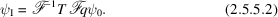International
Tables for
Crystallography
Volume B
Reciprocal space
Edited by U. Shmueli

International Tables for Crystallography (2010). Vol. B, ch. 2.5, p. 361   | 1 | 2 |

## Section 2.5.5.1. Introduction

B. K. Vainshteinc

#### 2.5.5.1. Introduction

| top | pdf |

In many fields of physical measurements, instrumental and informative techniques, including electron microscopy and computational or analogue methods for processing and transforming signals from objects investigated, find a wide application in obtaining the most accurate structural data. The signal may be radiation from an object, or radiation transmitted through the object, or reflected by it, which is transformed and recorded by a detector.

The image is the two-dimensional signalon the observation plane recorded from the whole three-dimensional volume of the object, or from its surface, which provides information on its structure. In an object this information may change owing to transformation of the scattered wave inside an instrument. The real imageis composed ofand noisefrom signal disturbances:Image-reconstruction methods are aimed at obtaining the most accurate information on the structure of the object; they are subdivided into two types (Picture Processing and Digital Filtering, 1975; Rozenfeld, 1969):

 (a) Image restoration – separation offrom the image by means of compensation of distortions introduced in it by an image-forming system as well as by an account of the available quantitative data reflecting its structure. (b) Image enhancement – maximum exclusion from the observed image(2.5.5.1)of all its imperfectionsfrom both accidental distortions in objects and various noise' in signals and detector, and obtainingas the result.

These two methods may be used separately or in combination.

The image should be represented in the form convenient for perception and analysis, e.g. in digital form, in lines of equal density, in points of different density, in half-tones or colour form and using, if necessary, a change or reversal of contrast.

Reconstructed images may be used for the three-dimensional reconstruction of the spatial structure of an object, e.g. of the density distribution in it (see Section 2.5.6).

This section is connected with an application of the methods of image processing in transmission electron microscopy (TEM). In TEM (see Section 2.5.2), the source-emitted electrons are transmitted through an object and, with the aid of a system of lenses, form a two-dimensional image subject to processing.

Another possibility for obtaining information on the structure of an object is structural analysis with the aid of electron diffraction – EDSA. This method makes use of information in reciprocal space – observation and measurement of electron-diffraction patterns and calculation from them of a two-dimensional projection or three-dimensional structure of an object using the Fourier synthesis. To do this, one has to find the relative phases of the scattered beams.

The wavefunction of an electron-microscopic image is written asHereis the incident plane wave. When the wave is transmitted through an object, it interacts with the electrostatic potential[is the three-dimensional vector in the space of the object]; this process is described by the Schrödinger equation (Section 2.5.2.1). As a result, on the exit surface of an object the wave takes the formwhere q is the transmission function and x is the two-dimensional vector. The diffraction of the waveis described by the two-dimensional Fourier operator:Here, we assume the initial wave amplitude to be equal to unity and the initial phase to be zero, so that, which defines, in this case, the wavefunction in the back focal plane of an objective lens with the reciprocal-space coordinates. The function Q is modified in reciprocal space by the lens transfer function. The scattered wave transformation into an image is described by the inverse Fourier operator.

The process of the diffraction, as seen from (2.5.5.1), is the same in both TEM and EDSA. Thus, in TEM under the lens actionsthe image formation from a diffraction pattern takes place with an account of the phases, but these phases are modified by the objective-lens transfer function. In EDSA, on the other hand, there is no distorting action of the transfer function and the image' is obtained by computing the operation.

The computation of projections, images and Fourier transformation is made by discretization of two-dimensional functions on a two-dimensional network of points – pixels in real spaceand in reciprocal space.

### References

Picture Processing and Digital Filtering (1975). Edited by T. S. Huang. Berlin: Springer-Verlag.
Rozenfeld, A. (1969). Picture Processing by Computer. New York: Academic Press.